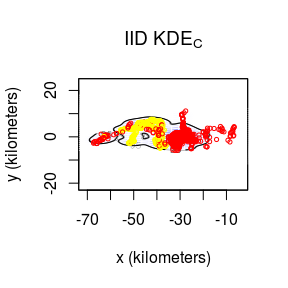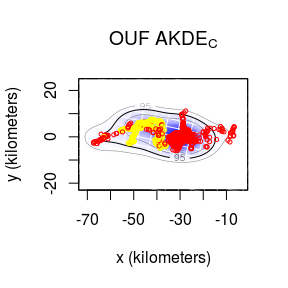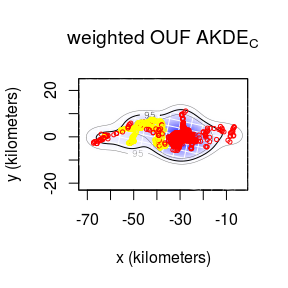# Autocorrelated Kernel Density Estimation

#### 2019-03-12

In this vignette we walk through autocorrelated kernel density estimation. We will assume that you have already estimated a good ctmm movement model for your data. For demonstration purposes we will be working from the results of the “Variograms and Model Selection” vignette (see vignette("variogram")). Here we will use the buffalo “Pepper” instead of “Cilla”, because Pepper has a GPS collar malfunction that can be mitigated with optimal weighting.

library(ctmm)
data(buffalo)
Pepper <- buffalo$Pepper M.IID <- ctmm.fit(Pepper) # no autocorrelation timescales m.ouf <- ctmm.guess(Pepper,interactive=FALSE) # automated model guess M.OUF <- ctmm.fit(Pepper,m.ouf) M.IID is the innapropriate, IID model, which will result in a conventional kernel-density estimate, while M.OUF is the superior, continuous-velocity OUF model. Note that you want the best model for each individual, even if that differs by individual. Different movement behaviors and sampling schedules will reveal different autocorrelation structures in the data. Now we can calculate an akde object for each model. UD0 <- akde(Pepper,M.IID) UD2 <- akde(Pepper,M.OUF) UD2w <- akde(Pepper,M.OUF,weights=TRUE) # calculate one extent for all UDs EXT <- extent(list(UD0,UD2,UD2w),level=0.95) Finally we calculate UDs with and with out accounting for autocorrelation (M.OUF versus M.IID), with and without optimal weighting of the data (weights=TRUE). Now let us plot the results. plot(Pepper,UD=UD0,xlim=EXT$x,ylim=EXT$y) title(expression("IID KDE"["C"])) plot(Pepper,UD=UD2,xlim=EXT$x,ylim=EXT$y) title(expression("OUF AKDE"["C"])) plot(Pepper,UD=UD2w,xlim=EXT$x,ylim=EXT$y) title(expression("weighted OUF AKDE"["C"]))By default both the density function and its 95% contours are plotted along with the location data. The middle contour represent the maximum likelihood area where the animal spends 95% of its time. This percentage can be changed with the level.UD option (see help(plot.telemetry)). The inner and outer contours correspond to confidence intervals on the magnitude of the area, which can be adjusted with the level option. The optimal bandwidth determines the “resolution” of the kernel density estimate. By default we plot grid lines with dimensions matching the standard deviations of the individual kernels. This gives a rough guideline as to what spatial details are and are not important in the density estimate. One can see that the IID KDEC estimate fits tightly to the data and reports many significant details in the buffalo’s home range. The autocorrelated estimates predict future space use more accurately, based on the diffusion model, and yield a more honest account of their uncertainties. In the case of Pepper, the GPS collar malfunctioned during data collection and the sampling interval mysteriously changed from 1 hour (yellow) to 2 hours (red). This irregularity makes Pepper a good candidate for optimally weighted AKDE (weights=TRUE). The optimally weighted estimate features smaller error, finer resolution, and mitigation of sampling bias. You can see that the uniformly weighted estimates place excessive emphasis on the oversampled data (yellow). Finally, we can compare the area estimates and effective sample sizes. summary(UD0) ##$DOF
##      area bandwidth
##      1725      1725
##
## $CI ## low ML high ## area (square kilometers) 436.6782 458.0407 479.9062 summary(UD2w) ##$DOF
##      area bandwidth
##  20.02345  31.67125
##
## \$CI
##                               low      ML     high
## area (square kilometers) 444.6052 727.639 1079.259